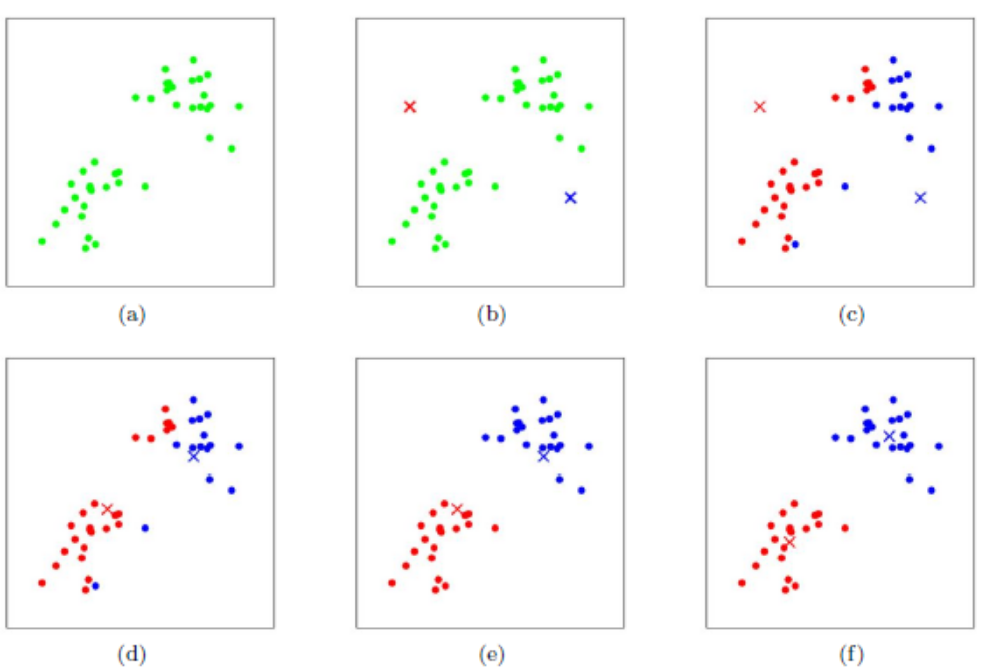# 经典分类算法

Posted by MaggicQ on February 25, 2019

# 经典分类算法

## 感知机

• 感知机是根据输入实例的特征向量$x$对其进行二类分类的线性分类模型：

• 感知机学习的策略是极小化损失函数：

• 感知机学习算法是基于随机梯度下降法的对损失函数的最优化算法，有原始形式和对偶形式。算法简单且易于实现。原始形式中，首先任意选取一个超平面，然后用梯度下降法不断极小化目标函数。在这个过程中一次随机选取一个误分类点使其梯度下降。

• 当训练数据集线性可分时，感知机学习算法是收敛的。当训练数据集线性可分时，感知机学习算法存在无穷多个解，其解由于不同的初值或不同的迭代顺序而可能有所不同。

### K-近邻算法

• $k$近邻法是基本且简单的分类与回归方法。$k$近邻法的基本做法是：对给定的训练实例点和输入实例点，首先确定输入实例点的$k$个最近邻训练实例点，然后利用这$k$个训练实例点的类的多数来预测输入实例点的类。
• $k$近邻模型对应于基于训练数据集对特征空间的一个划分。$k$近邻法中，当训练集、距离度量、$k$值及分类决策规则确定后，其结果唯一确定。
• $k$近邻法三要素：距离度量$k$值的选择分类决策规则常用的距离度量是欧氏距离及更一般的$pL$距离。$k$值小时，$k$近邻模型更复杂；$k$值大时，$k$近邻模型更简单。$k$值的选择反映了对近似误差与估计误差之间的权衡，通常由交叉验证选择最优的$k$。常用的分类决策规则是多数表决对应于经验风险最小化。
• $k$近邻法的实现需要考虑如何快速搜索$k$个最近邻点。$kd$树是一种便于对$k$维空间中的数据进行快速检索的数据结构。$kd$树是二叉树，表示对$k$维空间的一个划分，其每个结点对应于$k$维空间划分中的一个超矩形区域。利用$kd$树可以省去对大部分数据点的搜索，从而减少搜索的计算量。

## 朴素贝叶斯

### 数学推导

（注意上式中的分母，对不同的$Y = c_k$，其大小是相同的，所以要使后验概率最大，只要最大化它的分子就行了）

## 支持向量机

### 原理

SVM的机理是寻找一个满足分类要求的最优分类超平面，使得该超平面在保证分类精度的同时能够使超平面两侧的空白区域最大化。理论上，支持向量机能够实现对线性可分数据的最优分类

### 要点

1. 支持向量机最简单的情况是线性可分支持向量机，或硬间隔支持向量机。构建它的条件是训练数据线性可分。其学习策略是最大间隔法。可以表示为凸二次规划问题，其原始最优化问题为

1. 现实中训练数据是线性可分的情形较少，训练数据往往是近似线性可分的，这时使用线性支持向量机，或软间隔支持向量机。线性支持向量机是最基本的支持向量机。

对于噪声或例外，通过引入松弛变量${\varepsilon_i}$ ，使其“可分”，得到线性支持向量机学习的凸二次规划问题。

2. 对于输入空间中的非线性分类问题，可以通过非线性变换将它转化为某个高维特征空间中的线性分类问题，在高维特征空间中学习线性支持向量机。由于在线性支持向量机学习的对偶问题里，目标函数和分类决策函数都只涉及实例与实例之间的内积，所以不需要显式地指定非线性变换，而是用核函数来替换当中的内积。核函数表示，通过一个非线性转换后的两个实例间的内积。

具体地，$K(x, z)$是一个核函数，或正定核，意味着存在一个从输入空间$\mathcal{X}$的映射$\Phi(x): \mathcal{X} \rightarrow \mathcal{H}$

对任意$x, z \in \mathcal{X}$，有

1. $SMO$算法是支持向量机学习的一种快速算法，其特点是不断地将原二次规划问题分解为只有两个变量的二次规划子问题，并对子问题进行解析求解，直到所有变量满足$KKT$条件为止。这样通过启发式的方法得到原二次规划问题的最优解。因为子问题有解析解，所以每次计算子问题都很快，虽然计算子问题次数很多，但在总体上还是高效的。

### 拓展

SVM算法最初是为二值分类问题设计的，当处理多类问题时，就需要构造合适的多类分类器。

• 一对多法（one-versus-rest,简称OVR SVMs），训练时依次把某个类别的样本归为一类,其他剩余的样本归为另一类，这样k个类别的样本就构造出了k个SVM。分类时将未知样本分类为具有最大分类函数值的那类。这种方法有种缺陷,因为训练集是1:M,这种情况下存在biased，因而不是很实用。
• 一对一法（one-versus-one,简称OVO SVMs或者pairwise），在任意两类样本之间设计一个SVM，因此k个类别的样本就需要设计k(k-1)/2个SVM，当对一个未知样本进行分类时，使用k(k-1)/2个SVM分别对它进行分类，最后得票最多的类别即为该未知样本的类别。

## 决策树

• 特征选择
• 决策树的生成
• 决策树的修剪

### 特征选择

#### 信息增益

1. 计算数据集$D$的经验熵$H(D)$
1. 计算特征$A$对数据集$D$的经验条件熵$H(D|A)$
1. 计算信息增益

### C4.5的生成算法

1. 如果$D$中所有实例属于同一类$C_k$，则置$T$为单结点树，并将$C_k$作为该结点的类，返回$T$；
2. 如果$A$=Ø，则置$T$为单结点树，并将$D$中实例数最大的类$C_k$作为该结点的类，返回$T$；
3. 否则，计算$A$中各特征对$D$的信息增益比，选择信息增益比最大的特征$A_g$；
4. 如果$A$ 的信息增益比小于阈值$\gamma$ ，则置$T$为单结点树，并将$D_g$中实例数最大的类$C$ 作为该结点的类，返回$T$；
5. 否则，对$A_g$的每一可能值$a_i$，依$A_g$=$a_i$将$D$分割为子集若干非空$D_i$，将$D_i$中实例数最大的类作为标记，构建子结点，由结点及其子结点构成树$T$，返回$T$；
6. 对结点$i$，以$D_i$为训练集，以$A-{A_g}$为特征集，递归地调用1-5步，得到子树$T_i$，返回$T_i$。

## Ｋ均值算法(K-means)

K-Means算法是无监督的聚类算法，它实现起来比较简单，聚类效果也不错，因此应用很广泛。

### 原理

K-Means算法的思想很简单，对于给定的样本集，按照样本之间的距离大小，将样本集划分为K个簇。让簇内的点尽量紧密的连在一起，而让簇间的距离尽量的大。

K-Means采用的启发式方式很简单，用下面一组图就可以形象的描述：### 算法流程

1. 从数据集$D$中随机选择$k$个样本作为初始的$k$个质心向量：${\mu_1,\mu_2,…,\mu_k}$

2. 对于$n = 1,2, …, N$

1. 将簇划分C初始化为$C_t = \varnothing ; t =1,2…k$

2. 对于$i = 1,2,…m$，计算样本$x_i$和各个质心向量$\mu_j(j=1,2,…k)$的距离：$d_{ij} = ||x_i - \mu_j||_2^2$

将$x_i$标记最小的为$d_{ij}$所对应的类别$\lambda_i$。此时更新$C_{\lambda_i} = C_{\lambda_i} \cup {x_i}$

3. 对于$j=1，2，…，k$，对$C_j$中所有的样本点重新计算新的质心$\mu_j = \frac{1}{|C_j|}\sum\limits_{x \in C_j}x$

4. 如果所有的$k$个质心向量都没有发生变化，则转到步骤3

3. 输出簇划分$C=\{C_1,C_2,...C_k\}$

### 优缺点

K-Means的主要优点有：

• 原理比较简单，实现也是很容易，收敛速度快。
• 聚类效果较优。
• 算法的可解释度比较强。
• 主要需要调参的参数仅仅是簇数$k$。

• K值的选取不好把握
• 对于不是凸的数据集比较难收敛
• 如果各隐含类别的数据不平衡，比如各隐含类别的数据量严重失衡，或者各隐含类别的方差不同，则聚类效果不佳。
• 采用迭代方法，得到的结果只是局部最优。
• 对噪音和异常点比较的敏感。
• 计算量很大，要计算所有的样本点到所有的质心的距离。如果样本量非常大，比如达到10万以上，特征有100以上，此时用传统的K-Means算法非常的耗时。此时可以使用Mini Batch K-Means，也就是用样本集中的一部分的样本来做传统的K-Means，这样可以避免样本量太大时的计算难题，算法收敛速度大大加快。当然此时的代价就是我们的聚类的精确度也会有一些降低。一般来说这个降低的幅度在可以接受的范围之内。

# 常见问题

## SVM如何拓展到多分类？

• 一对多法（one-versus-rest,简称OVR SVMs），训练时依次把某个类别的样本归为一类,其他剩余的样本归为另一类，这样k个类别的样本就构造出了k个SVM。分类时将未知样本分类为具有最大分类函数值的那类。这种方法有种缺陷,因为训练集是1:M,这种情况下存在biased，因而不是很实用。
• 一对一法（one-versus-one,简称OVO SVMs或者pairwise），在任意两类样本之间设计一个SVM，因此k个类别的样本就需要设计k(k-1)/2个SVM，当对一个未知样本进行分类时，使用k(k-1)/2个SVM分别对它进行分类，最后得票最多的类别即为该未知样本的类别。

## SVM和逻辑回归的比较

• LR和SVM都是分类算法；
• 如果不考虑核函数，LR和SVM都是线性分类算法，也就是说他们的分类决策面都是线性的；
• LR和SVM都是监督学习算法；
• LR和SVM都是判别模型；
• LR和SVM在学术界和工业界都广为人知并且应用广泛；

• 本质上是其loss function不同；
• 支持向量机只考虑局部的边界线附近的点，而逻辑回归考虑全局（远离的点对边界线的确定也起作用）；
• 在解决非线性问题时，支持向量机采用核函数的机制，而LR通常不采用核函数的方法；
• 线性SVM依赖数据表达的距离测度，所以需要对数据先做normalization，LR不受其影响；
• SVM的损失函数就自带正则，这就是为什么SVM是结构风险最小化算法的原因，而LR必须另外在损失函数上添加正则项；

K-Means聚类算法原理